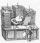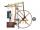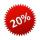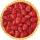# Arble bag

A marble bag sold by Rachel's Marble Company contains 5 orange marbles for every 6 green marbles. If a bag has 35 orange marbles, how many green marbles does it contain?

Result

g =  42

#### Solution:Leave us a comment of example and its solution (i.e. if it is still somewhat unclear...):

Showing 0 comments:Be the first to comment!## Next similar examples:

1. GlovesI have a box with two hundred pieces of gloves in total, split into ten parcels of twenty pieces, and I sell three parcels. What percent of the total amount I sold?
2. BureaucracyThe office was attended by 23 people. 9 of them completed the questionnaire. What is the percentage of all people?
3. Ratio v2Decrease in the ratio 12:16 number 13.2.
4. RatioIncrease in the ratio 20:4 number 18.5.
5. EfficiencyA certain device consumes 28 kW and delivers 14 kW. Find its efficiency.
6. Two numbersFind two numbers whose difference and ratio is 2.
7. Percentages and numbersHow many percent is number 426 greater than number 71?
8. Strange xFor what x is true ??
9. 1.5 divided1.5 divided by 1 = w divided by 4
10. DonutsFind how many donuts each student will receive if you share 126 donuts in a ratio of 1:5:8
11. TVsProduction of television sets increased from 3,500 units to 4,200 units. Calculate the percentage of production increase.
12. Down syndromeDown syndrome is one of the serious diseases caused by a gene mutation. Down syndrome occurs in approximately every 550-born child. Express the incidence of Down's syndrome in newborns at per mille.
13. Base, percents, valueBase is 344084 which is 100 %. How many percent is 384177?
14. InequationSolve the inequation: 5k - (7k - 1)≤ 2/5 . (5-k)-2
15. Apples 2James has 13 apples. He has 30 percent more apples than Sam. How many apples has Sam?
16. Sales offGoods is worth € 70 and the price of goods fell two weeks in a row by 10%. How many % decreased overall?
17. EquationSolve the equation: 1/2-2/8 = 1/10; Write the result as a decimal number.